moveit2 The MoveIt Motion Planning Framework for ROS 2.
stomp_moveit::costs Namespace Reference

## Functions

CostFn getCostFunctionFromStateValidator (const StateValidatorFn &state_validator_fn, double interpolation_step_size)

CostFn getCollisionCostFunction (const std::shared_ptr< const planning_scene::PlanningScene > &planning_scene, const moveit::core::JointModelGroup *group, double collision_penalty)

CostFn getConstraintsCostFunction (const std::shared_ptr< const planning_scene::PlanningScene > &planning_scene, const moveit::core::JointModelGroup *group, const moveit_msgs::msg::Constraints &constraints_msg, double cost_scale)

CostFn sum (const std::vector< CostFn > &cost_functions)

## Variables

constexpr double COL_CHECK_DISTANCE = 0.05

constexpr double CONSTRAINT_CHECK_DISTANCE = 0.05

## ◆ getCollisionCostFunction()

 CostFn stomp_moveit::costs::getCollisionCostFunction ( const std::shared_ptr< const planning_scene::PlanningScene > & planning_scene, const moveit::core::JointModelGroup * group, double collision_penalty )

Creates a cost function for binary collisions of group states in the planning scene. This function uses a StateValidatorFn for computing smooth penalty costs from binary collision checks using getCostFunctionFromStateValidator().

Parameters
 planning_scene The planning scene instance to use for collision checking group The group to use for computing link transforms from joint positions collision_penalty The penalty cost value applied to colliding states
Returns
Cost function that computes smooth costs for colliding path segments

Definition at line 168 of file cost_functions.hpp.

Here is the call graph for this function: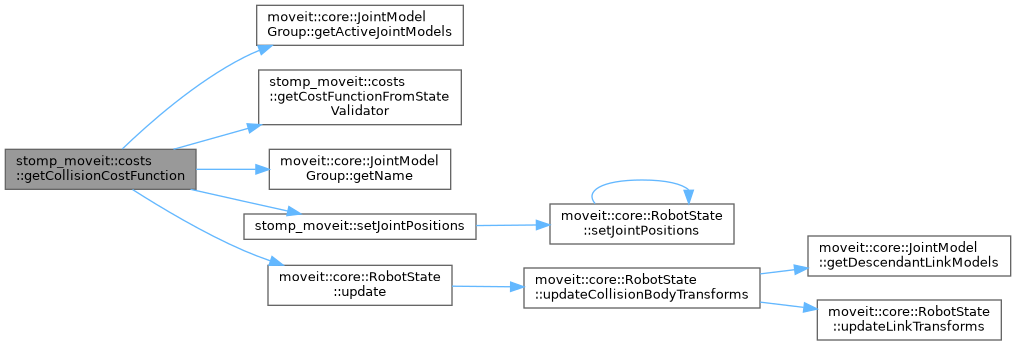Here is the caller graph for this function: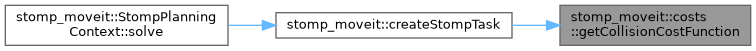## ◆ getConstraintsCostFunction()

 CostFn stomp_moveit::costs::getConstraintsCostFunction ( const std::shared_ptr< const planning_scene::PlanningScene > & planning_scene, const moveit::core::JointModelGroup * group, const moveit_msgs::msg::Constraints & constraints_msg, double cost_scale )

Creates a cost function for binary constraint checks applied to group states. This function uses a StateValidatorFn for computing smooth penalty costs from binary constraint checks using getCostFunctionFromStateValidator().

Parameters
 planning_scene The planning scene instance to use for computing transforms group The group to use for computing link transforms from joint positions constraints_msg The constraints used for validating group states cost_scale A scalar factor applied to the distance cost of invalid states
Returns
Cost function that computes smooth costs for invalid path segments

Definition at line 199 of file cost_functions.hpp.

Here is the call graph for this function: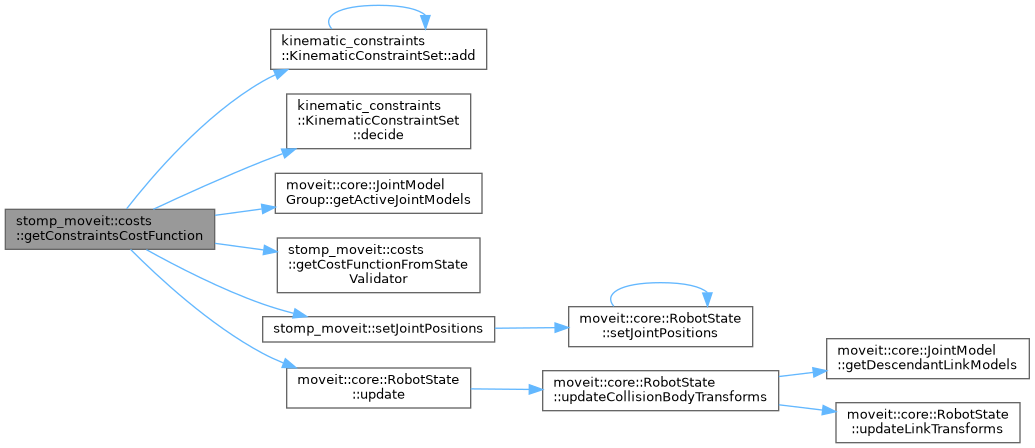Here is the caller graph for this function: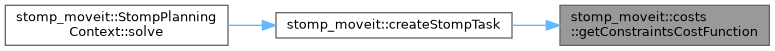## ◆ getCostFunctionFromStateValidator()

 CostFn stomp_moveit::costs::getCostFunctionFromStateValidator ( const StateValidatorFn & state_validator_fn, double interpolation_step_size )

Creates a cost function from a binary robot state validation function. This is used for computing smooth cost profiles for binary state conditions like collision checks and constraints. The validator function is applied for all states in the validated path while also considering interpolated states. If a waypoint or an interpolated state is invalid, a local penalty is being applied to the path. Penalty costs are being smoothed out using a Gaussian so that valid neighboring states (near collisions) are optimized as well.

Parameters
 state_validator_fn The validator function that tests for binary conditions interpolation_step_size The L2 norm distance step used for interpolation
Returns
Cost function that computes smooth costs for binary validity conditions

Definition at line 75 of file cost_functions.hpp.

Here is the caller graph for this function: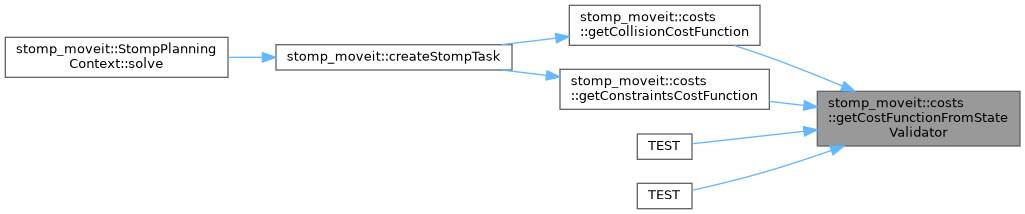## ◆ sum()

 CostFn stomp_moveit::costs::sum ( const std::vector< CostFn > & cost_functions )

Creates a cost function that computes the summed waypoint penalites over a vector of cost functions.

Parameters
 cost_functions A vector of cost functions
Returns
Cost function that computes the summed costs for each waypoint

Definition at line 228 of file cost_functions.hpp.

Here is the caller graph for this function: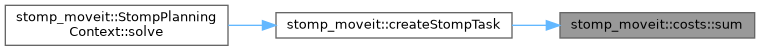## ◆ COL_CHECK_DISTANCE

 constexpr double stomp_moveit::costs::COL_CHECK_DISTANCE = 0.05
constexpr

Definition at line 59 of file cost_functions.hpp.

## ◆ CONSTRAINT_CHECK_DISTANCE

 constexpr double stomp_moveit::costs::CONSTRAINT_CHECK_DISTANCE = 0.05
constexpr

Definition at line 60 of file cost_functions.hpp.